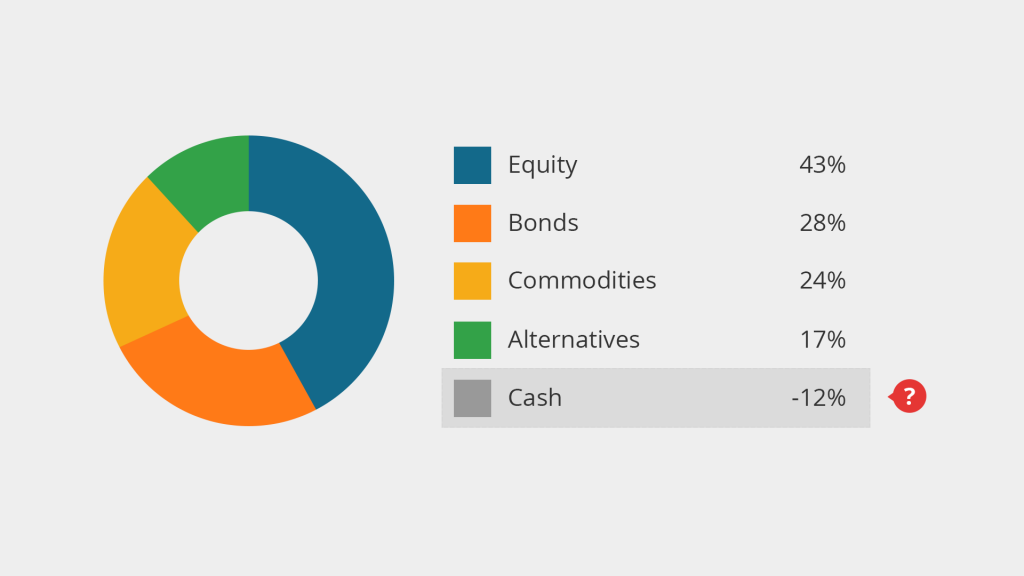### Negative numbers in the asset allocation

The asset allocation is one of the most important components of a portfolio reporting as it represents the individual investment strategy. The percentage distribution of e.g. stocks, bonds, commodities and cash indicates the level of risk and thus the expected return.

Most of our customers use asset allocations within their factsheets. Usually the asset class for each security is assigned individually from the portfolio managers to make sure the factsheet precisely represents the investment strategy. The asset allocation normally sums up to 100%, meaning that the invested volume and the cash available add up to the fund’s total net assets under management.

Shorting an asset, e.g. a share, can cause allocation statistics to get negative. Imagine a Long-Short strategy with two classifications: “Equity Long” and “Equity Short”. For sure, the asset allocation weighting of the “Equity Short” classification will be negative as all short investments are allocated under this classification.

### The impact of derivatives

But there is another portfolio construction which makes weightings of asset classes to appear negative. In the following paragraphs we will show how the use of derivatives, more concretely of futures contracts, can make your cash weighting negative. This has to do with the leverage of those futures and the resulting difference between the exposure and the actual book value of those contracts. So let’s first take a minute to explain the characteristics of those derivatives.

Derivatives in general derive their value from the performance of an underlying, e.g. an index, share or commodity. The exposure of those derivatives to the price movements of the underlying can be modified almost arbitrarily by the issuer. The participation in the market value changes of the underlying can be weaker or stronger (leverage effect) or even be inverse. A small difference in the market value of the underlying can cause a large difference in the value of the derivative.

A very common form of derivatives are futures. These contractual agreements describe the purchase or sale of a financial instrument at a pre-determined price in the future. The buyer of a futures contract is said to go long, the selling party is said go short. Futures are traded on exchanges which decreases the counterparty risk to almost zero. To ensure that traders of a futures contract can at any time cover potential losses they need to pay a certain amount of the overall exposure of that futures contract into a margin account. In case the futures’ price moves unfavourably the trader needs to cover the losses occurring on this margin account (margin call), on the other hand if the price of the futures contract moves in a favourable direction the trader is allowed to withdraw the profit made from that account.

Thus, as the trader only puts a fraction of the overall futures contract exposure in cash on the table, there is leverage. E.g. let’s assume a trader enters a futures contract with an overall exposure in the underlying of 100,000 EUR and the requested margin of the exchange for that trade, the booking value of that futures contract, is 20,000 EUR. A 1% increase in the underlying (+1,000 EUR) would result in a 5% increase in the booking value of that futures contract. The leverage in this example is thus 5.

Now after understanding the basic mechanism of futures and the terminology, let’s have a look at a portfolio example.

### Portfolio Example

Let’s assume we manage a fund with overall 500,000 EUR net assets under management. The total volume is available in cash, so the portfolio allocation is 100% cash. Now let’s enter into 10 of the futures contracts described above. The portfolio would now be left with 300,000 EUR in cash and 200,000 EUR invested in the above futures contract, thus 60% cash and 40% futures.

Is this the most accurate representation? Looking at the booking value, like most standard reporting solutions do, this allocation is mathematically correct. But every fund manager would agree that the allocation shown based on the booking value of the futures is useless in almost every case. As the 200,000 EUR booking value actually represents an exposure in the underlying of 1,000,000 EUR the allocation should actually show 60% cash and 200% underlying. This would reflect the actual risk and expected potential return of our portfolio.

There is only one challenge left now. While the sum of all allocation positions in the calculation using the booking value equals 100%, as expected, the sum using the interpretable exposure does not. With 260% it is actually quite significantly above the 100% and thus would raise a lot of questions from investors. From a financial point of view the increased exposure of the futures contract compared to the booking value is based on the leverage. To gain the same exposure investing in the underlying and not in the futures contract without using the remaining cash would require lending cash, thus going short in cash, and investing this cash as well in the underlying. So the most widely used procedure to solve the above issue of an overall allocation of more than 100% is balancing the leverage by an additional position called “synthetic cash”.

In our example this would result in an allocation of 60% cash, 200% underlying and -160% synthetic cash. As asset managers want to keep statistics as clear as possible these two cash positions are unified. The resulting allocation looks like this:

200% underlying, -100% cash (incl. synthetic cash)

And this is how an asset allocation can show negative numbers even without shorting.

WEBINAR